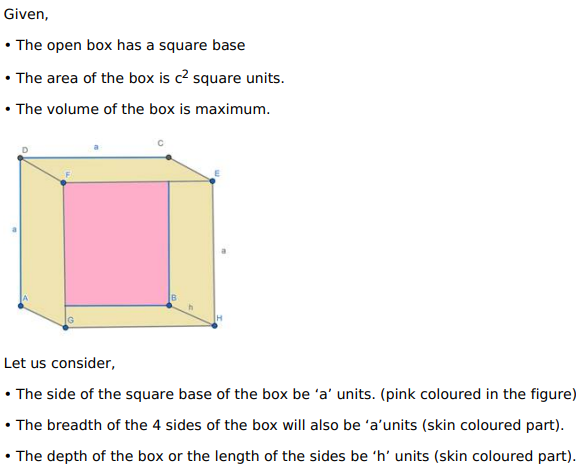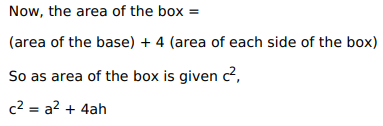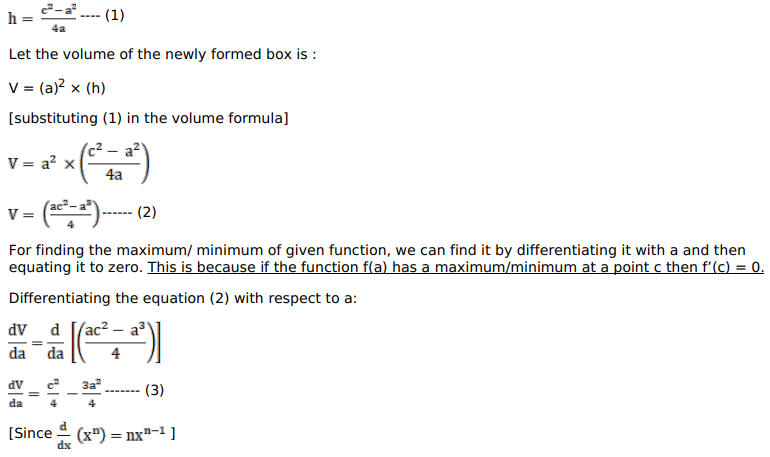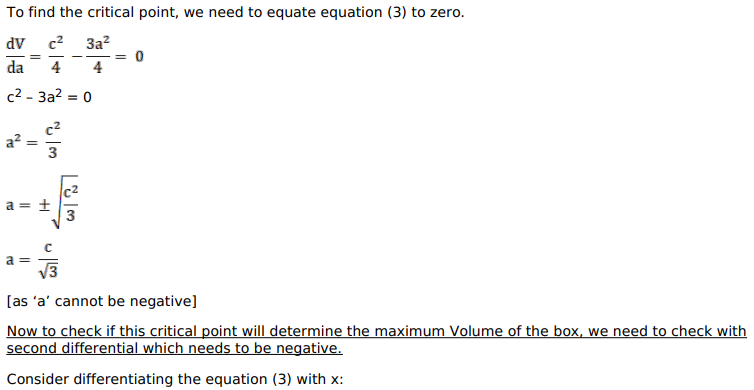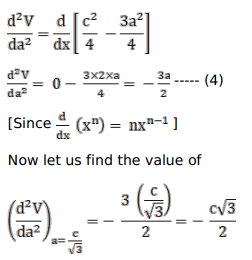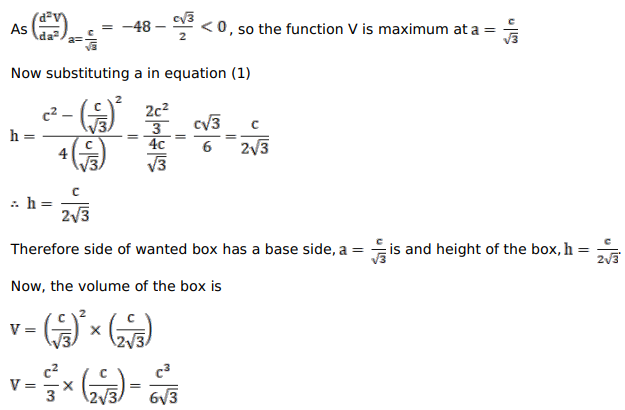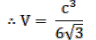# An open box with a square base is to be made out of a given cardboard

Question:

An open box with a square base is to be made out of a given cardboard of area $\mathrm{c}^{2}$ (square) units. Show that the maximum volume of the box is $\frac{\mathrm{c}^{3}}{6 \sqrt{3}}$ (cubic) units.

Solution: